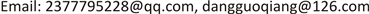﻿ 亚纯函数分担集合的正规定则 Normality Concerning Meromorphic Functions and Shared Set

Pure Mathematics
Vol. 09  No. 04 ( 2019 ), Article ID: 30979 , 6 pages
10.12677/PM.2019.94069

Normality Concerning Meromorphic Functions and Shared Set

Jinhua Cai, Guoqiang Dang

School of Mathematics and Information Science, Guangzhou University, Guangzhou GuangdongReceived: May 31st, 2019; accepted: Jun. 10th, 2019; published: Jun. 26th, 2019ABSTRACT

The normal family of meromorphic functions concerning shared set was studied. The following result was proved: Let $F$ be a family of meromorphic functions in a domain D, a, b and c be three finite complex numbers, where $a\ne b$, k and q be two positive integers, let $S=\left\{a,b\right\}$. If for each $f\in F$, 1) $\forall z\in D$$h\left({f}^{\left(k\right)}\left(z\right)\right)\in S⇒f\left(z\right)\in S$, where ${a}_{i}\left(z\right)\left(i=1,2,\cdots ,q-1\right)$ is holomorphic function and $h\left({f}^{\left(k\right)}\left(z\right)\right)={\left({f}^{\left(k\right)}\left(z\right)\right)}^{q}+{a}_{q-1}\left(z\right){\left({f}^{\left(k\right)}\left(z\right)\right)}^{q-1}+\cdots +{a}_{1}\left(z\right){f}^{\left(k\right)}\left(z\right)$; 2) $f-c$ have zeros with multiplicities at least $k+1$, then $F$ is normal in D.

Keywords:Meromorphic Functions, Normality, Shared Set1. 引言及主要结果

$F$ 为区域D内的一族亚纯函数，如果对于 $F$ 的任一函数序列 $\left\{{f}_{n}\left(z\right)\right\}$ 均可选出一个子序列 $\left\{{f}_{nj}\left(z\right)\right\}$ 在区域D上按球距内闭一致收敛，则称 $F$ 在D内正规。

1992年，Schwick  首次把亚纯函数正规族与分担值联系起来，证明了

2008年，韩明华和顾永兴  探讨 ${f}^{\left(k\right)}$ 和f分担两个值的情形，证明了

2018年，陈鸿辉等人  考虑 ${\left({f}^{\left(k\right)}\right)}^{q}$ 和f分担一个集合S的情况，证明了

2. 引理

1) 函数列 ${f}_{n}\in F$

2) 点列 ${z}_{n}\in D$${z}_{n}\to {z}_{0}$

3) 正数列 ${\rho }_{n}\to 0$

4) 实数r， $0

1) $n=k,n!{a}_{n}=1$

2) $f\left(z\right)=\frac{{z}^{k}}{k!}+\cdots +{a}_{1}+{a}_{0}+\frac{1}{{\left(az+b\right)}^{m}}$，其中 $a\left(\ne 0\right)$ 和b是常数；

3) 若 $f\left(z\right)$ 的零点重级均 $\ge k+1$，则结论2) 中 $m=1$，且 $f\left(z\right)=\frac{{\left(cz+d\right)}^{k+1}}{az+b}$，其中 $a\left(\ne 0\right),b$ 和d是常数。

3. 定理1的证明

1) $h\left({g}^{\left(k\right)}\left(\xi \right)\right)\ne a$

2) $h\left({g}^{\left(k\right)}\left(\xi \right)\right)\ne b$

${g}_{n}\left(\xi \right)=\frac{{f}_{n}\left({z}_{n}+{\rho }_{n}\xi \right)-c}{{\rho }_{n}{}^{k}}\to g\left(\xi \right)$

${g}_{n}^{\left(k\right)}\left(\xi \right)={f}_{n}^{\left(k\right)}\left({z}_{n}+{\rho }_{n}\xi \right)\to {g}^{\left(k\right)}\left(\xi \right)$

${\left({g}_{n}^{\left(k\right)}\left(\xi \right)\right)}^{q}={\left({f}_{n}^{\left(k\right)}\left({z}_{n}+{\rho }_{n}\xi \right)\right)}^{q}\to {\left({g}^{\left(k\right)}\left(\xi \right)\right)}^{q}$

$\begin{array}{l}h\left({f}_{n}^{\left(k\right)}\left({z}_{n}+{\rho }_{n}\xi \right)\right)-a\\ ={\left({f}^{\left(k\right)}\left({z}_{n}+{\rho }_{n}\xi \right)\right)}^{q}+{a}_{q-1}\left({z}_{n}+{\rho }_{n}\xi \right){\left({f}^{\left(k\right)}\left({z}_{n}+{\rho }_{n}\xi \right)\right)}^{q-1}+\cdots +{a}_{1}\left({z}_{n}+{\rho }_{n}\xi \right){f}^{\left(k\right)}\left({z}_{n}+{\rho }_{n}\xi \right)-a\\ ={\left({g}^{\left(k\right)}\left({z}_{n}+{\rho }_{n}\xi \right)\right)}^{q}+{a}_{q-1}\left({z}_{n}+{\rho }_{n}\xi \right){\left({g}^{\left(k\right)}\left({z}_{n}+{\rho }_{n}\xi \right)\right)}^{q-1}+\cdots +{a}_{1}\left({z}_{n}+{\rho }_{n}\xi \right){g}^{\left(k\right)}\left({z}_{n}+{\rho }_{n}\xi \right)-a\\ =h\left({g}_{n}^{\left(k\right)}\left({z}_{n}+{\rho }_{n}\xi \right)\right)-a\end{array}$

3) $h\left({g}^{\left(k\right)}\left(\xi \right)\right)=a⇒g\left(\xi \right)=0$

4) $h\left({g}^{\left(k\right)}\left(\xi \right)\right)=b⇒g\left(\xi \right)=0$

Normality Concerning Meromorphic Functions and Shared Set[J]. 理论数学, 2019, 09(04): 527-532. https://doi.org/10.12677/PM.2019.94069

1. 1. Schwick, W.K. (1992) Sharing Values and Normality. Archiv der Mathematik, 59, 50-54.
https://doi.org/10.1007/BF01199014

2. 2. Fang, M.L. and Zalcman, L. (2004) A Note on Normality and Shared Values. Journal of the Australian Mathematical Society, 76, 141-150.
https://doi.org/10.1017/S1446788700008752

3. 3. Fang, M.L. and Zalcman, L. (2002) Normal Families and Shared Values of Meromorphic Function III. Computational Methods and Function Theory, 2, 385-395.
https://doi.org/10.1007/BF03321856

4. 4. Pang, X.C. and Zalcman, L. (2000) Normality and Shared Values. Arkiv för Matematik, 38, 171-182.
https://doi.org/10.1007/BF02384496

5. 5. 刘晓俊, 庞学诚. 分担值与正规族[J]. 数学学报, 2007, 52(2): 409-412.

6. 6. 张汉, 谢东, 张庆德. 涉及分担集的亚纯函数的正规定则[J]. 数学物理学报, 2011, 31(5): 1290-1294.

7. 7. Han, M.H. and Gu, Y.X. (2008) The Normal Family of Mermorphic Functions. Acta Mathematica Scientia, 28, 759-762.
https://doi.org/10.1016/S0252-9602(08)60076-4

8. 8. 陈鸿辉, 蔡金华, 袁文俊. 涉及分担集合的亚纯函数正规定则[J]. 嘉应学院学报(自然科学), 2018, 36(11): 5-8.

9. 9. Zalcman, L. (1998) Normal Families: New Perspec-tives. Bulletin of the American Mathematical Society, 35, 215-230.
https://doi.org/10.1090/S0273-0979-98-00755-1

10. 10. Bergweiler, W. and Eremanko, A. (1995) On the Singularities of the Inverse to a Meromorphic Functions of Finite Order. Revista Matemática Iberoamericana, 11, 355-373.
https://doi.org/10.4171/RMI/176

11. 11. 顾永兴, 庞学诚, 方明亮. 正规族理论及其应用[M]. 北京: 科学出版社, 2007: 62-64.

12. 12. Fang, M.L. and Zalcman, L. (2003) Normal Families and Shared Values of Meromorphic Functions. An-nales Polonici Mathematici, 80, 137-141.
https://doi.org/10.4064/ap80-0-11